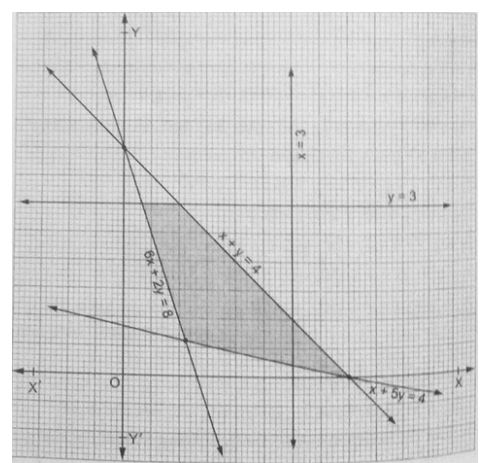# Find the linear inequalities for which the shaded area is the solution set in the figure given below.

Question:

Find the linear inequalities for which the shaded area is the solution set in the figure given below.Solution:

We have seen that the shaded region and origin are on the opposite side of the line $6 x+2 y=8$

For $(0,0)$ we have $0+0-8<0$. So the shaded region satisfies the inequality $6 x+2 y \geq 8$.

We have seen that the shaded region and origin are on the opposite side of the line $x+5 y=4$

For $(0,0)$ we have $0+0-4<0$. So the shaded region satisfies the inequality $x+5 y \geq 4$.

We have seen that the shaded region and origin are on the same side of the line $x+y=4$

For $(0,0)$ we have $0+0-4<0$. So the shaded region satisfies the inequality $\boldsymbol{x}+\boldsymbol{y} \leq \mathbf{4}$.

We have seen that the shaded region and origin are on the same side of the line $y=3$

For $(0,0)$ we have $0-3<0$. So the shaded region satisfies the inequality $y \leq 3$.

Thus the linear inequation comprising the given solution set are $+2 y \geq 8, x+5 y \geq 4, x+y \leq 4, y \leq 3$